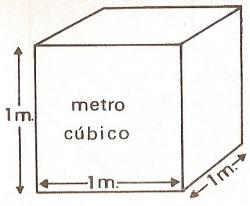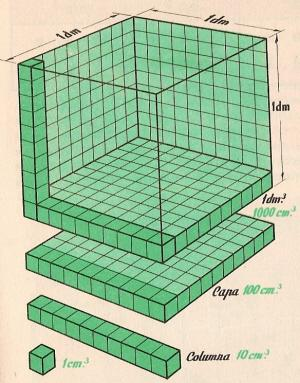Units of volume to printName: ______________________________________  Subject: _______________________ Date: _______

Write on the right side what is missing.

1. The cubic meter

The cubic meter is the volume of a cube with edges one meter in length. Its symbol is: m3.

2. Multiples of the cubic meter

These are:

1 cubic decameter is equal to 1 000 cubic meters: 1 dam3 = 1 000 m3 .
1 cubic hectometer is equal to 1 000 000 cubic meters: 1 hm3 = 1 000 000 m3.
1 cubic kilometer is equal to 1 000 000 000 cubic meters: 1 km3 = 1 000 000 000 m3.
1 cubic myriameter is equal to 1 000 000 000 000 cubic meters: 1 mam3 = 1 000 000 000 000 m3.

The units of volume increase and decrease from 1000 to 1000.
The superior unit is 1000 more than the lower.

It answers these questions in: m3:

 3 km3 = 7 dam3 = 8 hm3 =3. - Submultiples of the cubic meter

The picture shows a cube that has 1dm per side. Its volume is the unit called cubic decimeter (dm3). It can be divided into 10 layers of 100 cm3. Then 1 dm3 = 1000 cm3. Each cm3 can be divided into 1000 parts or mm3..

The submultiples are these:

1 cubic decimeter is equal to 0.001 cubic meter: 1 dm3 = 0,001 m3. 1 m3 tiene 1 000 dm3.
1 cubic centimeter is equal to 0.000 001 cubic meter: 1 cm3 = 0,000 001 m3. El m3 is 1 000 000 cm3.
1 cubic millimeter = 0.000 000 001cubic meter: 1 mm3 = 0,000 000 001 m3. El m3 is 1 000 000 000 m3.

The units of volume increase and decrease from 1000 to 1000.
The lower unit is 1000 less than the superior.

It answers these questions in m3:

 7 dm3 = 3 137 cm3 = 8 385 dm3 =

4. Conversion of unit

Each unit of volume is 1000 times greater than the immediate lower and 1000 times less than the immediate superior.

To convert from dam3 to m3 we multiply by 1000 or we move the decimal point three places to the right.
Examples: 5 dam3 = 5000 m3; 25,324 hm3 = 25 324 dam3 = 25 324 000 m3.

To convert from m3 to dam3 we divide by 1000 or we move the decimal point three places to the left.
Examples: 2 m3 = 0,002 dam3; 1 468 m3 = 1,468 dam3 = 0,001 468 hm3 = 0,000 001 468 km3.

It answers these questions in m3:

 7,32 dam3 = 18,457 hm3 = 0,0073 km3=

5. Converting from complex to non-complex

To convert from complex of volume to non-complex of lower order, we write the numbers of successive orders, reserving two places for each order and placing zeros in the empty places.

Example: 3 mam3, 735 hm3 y 5 cm3 can be written as: 3 000 735 000 000, 000 005 m3

It answers these questions in m3

 3 km3, 741 dam3 y 31 m3 = 83 hm3 y 798 dm3 = 7 dam3, 8 dm3 y 3 cm2 =

6. Converting from non-complex ( 12 500 m3 ) to complex ( 12 dam3 y 500 m3 ).

To convert from non-complex of volume to complex, we separate the numbers in groups of three, from the decimal point, putting the corresponding names to their orders. The number of the units is the same order as the incomplete.

For example: 4 023 715,67 cm3, can be written as: 4 m3, 23 dm3 and 715 cm3 y 670 mm3.

Examples:
32 000 026 m3 = 32 hm3  and 26 m3
17,028 m3 = 17 m3 and 28 dm3.
5,6 mam3 = 5 mam3 and 600 km3.

7. Problems

To find the volume of a cube, we multiply the length by the width by the height.

Do these problems on paper and write the solution:

 1. A cube is 4.5 cm of edge. How many cm3 does the volume is? 2. A die is 2 cm of edge. What is its volume in cm3? 3. The cubic pieces of soap of 5 cm edge has been send in cubic boxes of 60 cm edge . How many pieces can the box contain? 4. In a box of 0.696 dam3, how many cubes of 12 m3 fit? 5. There are 1.23 m3 of wine in a barrel. How many bottles of 0.75 liters can we fill? (1 liter=1 dm3) 6. A jar that has 0.4 m3 of oil has cost 800 euros. How much money do we have to pay for a liter? 7. A vintner buys 3 m3. First he sold 128 liters and the rest is distributed in 8 equal barrels. How many dm3 did he pour in each barrel? 8. A boat transports 75 dam3 of wine and we want pack it in barrels of 1.2 m3. How many barrels will we need? 9. A box is 3.5 m on each side. How many liters of water will fit in the box? 10. A candy has a volume of 1.3 cm3. How many candies fit in a box of 0.4498 dm3?

| Educational applications | Matemßticas |
In Spanish  | Interactive

«Arturo Ramo GarcĒa.-Record of intellectual property of Teruel (Spain) No 141, of 29-IX-1999
Plaza Playa de Aro, 3, 1║ DO 44002-TERUEL